A class to iterate over all permutations of an array. : Array « Collections Data Structure « Java

A class to iterate over all permutations of an array.

```
/*
* Version 1.0, and under the Eclipse Public License, Version 1.0
* Initial Developer: H2 Group
*
* According to a mail from Alan Tucker to Chris H Miller from IBM,
* the algorithm is in the public domain:
*
* Date: 2010-07-15 15:57
* Subject: Re: Applied Combinatorics Code
*
* Chris,
* The combinatorics algorithms in my textbook are all not under patent
* or copyright. They are as much in the public domain as the solution to any
* common question in an undergraduate mathematics course, e.g., in my
* combinatorics course, the solution to the problem of how many arrangements
* there are of the letters in the word MATHEMATICS. I appreciate your due
* diligence.
* -Alan
*/
//package org.h2.util;

//import org.h2.message.DbException;

/**
* A class to iterate over all permutations of an array.
* The algorithm is from Applied Combinatorics, by Alan Tucker as implemented in
* http://www.koders.com/java/fidD3445CD11B1DC687F6B8911075E7F01E23171553.aspx
*
* @param <T> the element type
*/
public class Permutations<T> {

private T[] in;
private T[] out;
private int n, m;
private int[] index;
private boolean hasNext = true;

private Permutations(T[] in, T[] out, int m) {
this.n = in.length;
this.m = m;
if (n < m || m < 0) {
//   DbException.throwInternalError("n < m or m < 0");
}
this.in = in;
this.out = out;
index = new int[n];
for (int i = 0; i < n; i++) {
index[i] = i;
}

// The elements from m to n are always kept ascending right to left.
// This keeps the dip in the interesting region.
reverseAfter(m - 1);
}

/**
* Create a new permutations object.
*
* @param <T> the type
* @param in the source array
* @param out the target array
* @return the generated permutations object
*/
public static <T> Permutations<T> create(T[] in, T[] out) {
return new Permutations<T>(in, out, in.length);
}

/**
* Create a new permutations object.
*
* @param <T> the type
* @param in the source array
* @param out the target array
* @param m the number of output elements to generate
* @return the generated permutations object
*/
public static <T> Permutations<T> create(T[] in, T[] out, int m) {
return new Permutations<T>(in, out, m);
}

/**
* Move the index forward a notch. The algorithm first finds the rightmost
* index that is less than its neighbor to the right. This is the dip point.
* The algorithm next finds the least element to the right of the dip that
* is greater than the dip. That element is switched with the dip. Finally,
* the list of elements to the right of the dip is reversed.
* For example, in a permutation of 5 items, the index may be {1, 2, 4, 3,
* 0}. The dip is 2 the rightmost element less than its neighbor on its
* right. The least element to the right of 2 that is greater than 2 is 3.
* These elements are swapped, yielding {1, 3, 4, 2, 0}, and the list right
* of the dip point is reversed, yielding {1, 3, 0, 2, 4}.
*/
private void moveIndex() {
// find the index of the first element that dips
int i = rightmostDip();
if (i < 0) {
hasNext = false;
return;
}

// find the least greater element to the right of the dip
int leastToRightIndex = i + 1;
for (int j = i + 2; j < n; j++) {
if (index[j] < index[leastToRightIndex] && index[j] > index[i]) {
leastToRightIndex = j;
}
}

// switch dip element with least greater element to its right
int t = index[i];
index[i] = index[leastToRightIndex];
index[leastToRightIndex] = t;

if (m - 1 > i) {
// reverse the elements to the right of the dip
reverseAfter(i);

// reverse the elements to the right of m - 1
reverseAfter(m - 1);
}
}

/**
* Get the index of the first element from the right that is less
* than its neighbor on the right.
*
* @return the index or -1 if non is found
*/
private int rightmostDip() {
for (int i = n - 2; i >= 0; i--) {
if (index[i] < index[i + 1]) {
return i;
}
}
return -1;
}

/**
* Reverse the elements to the right of the specified index.
*
* @param i the index
*/
private void reverseAfter(int i) {
int start = i + 1;
int end = n - 1;
while (start < end) {
int t = index[start];
index[start] = index[end];
index[end] = t;
start++;
end--;
}
}

/**
* Go to the next lineup, and if available, fill the target array.
*
* @return if a new lineup is available
*/
public boolean next() {
if (!hasNext) {
return false;
}
for (int i = 0; i < m; i++) {
out[i] = in[index[i]];
}
moveIndex();
return true;
}

}

```

Related examples in the same category

 1 Initialize a static array 2 Initialization and re-assignment of arrays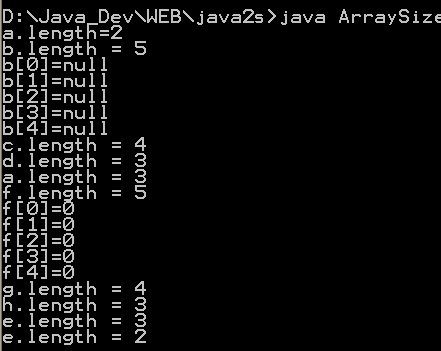3 Doubling the size of an array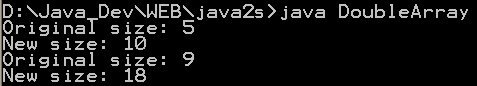4 Timing array loop performance 5 Array 2D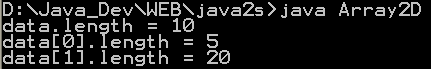6 Can you change the .length of an array 7 Show Two-Dimensional Array of Objects 8 ArrayListDemo done over using an ArrayList 9 Array Hunt game 10 Multi Dimension Array 11 Clone Array 12 Associates keys with values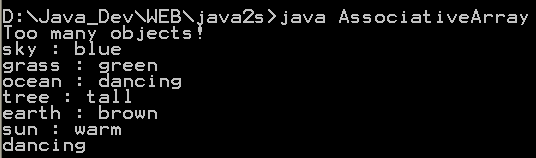13 Arrays of primitives 14 Creating arrays with new 15 Array initialization 16 Creating an array of nonprimitive objects 17 Create multidimension arrays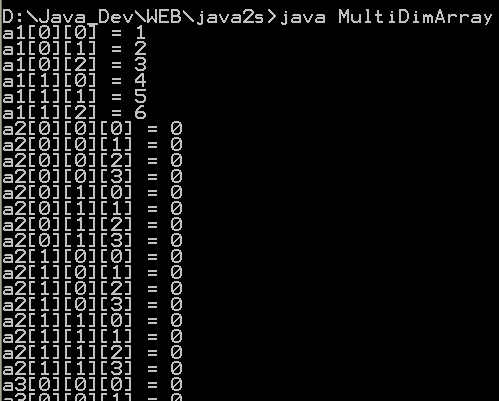18 Initializing Array Values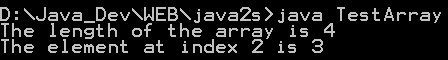19 Creating a Two-Dimensional Array 20 Initializing a Two Dimensional Array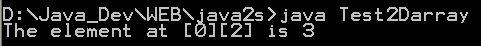21 Using the length Variable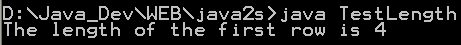22 Triangular array 23 Grow array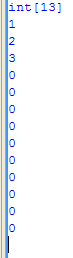24 Define array for class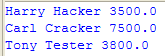25 String array and output to console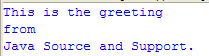26 Multiply two matrices 27 Array Of Arrays Demo 2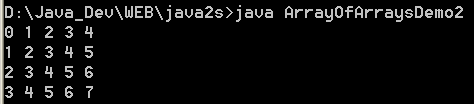28 Array Copy Demo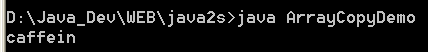29 Copying Elements from One Array to Another 30 Java program to demonstrate multidimensional arrays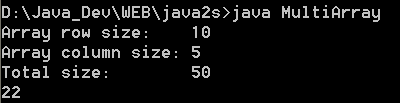31 Extend the size of an array 32 Copy an array 33 Initialize multidimensional array 34 Get array upperbound 35 To get the number of dimensions 36 Resize an array, System.arraycopy() 37 Dump array content: Convert the array to a List and then convert to String 38 java.utils.Arrays provides ways to dump the content of an array. 39 Dump multi-dimensional arrays 40 Use the new shorthand notation to iterate through an array 41 Create a repeated sequence of character 42 Reverse array elements order 43 Convert array of primitives into array of objects 44 Array Initializers 45 Reinitializes a byte array 46 Reinitializes an int array 47 Sum all elements in the array 48 Sums an array of numbers log(x1)...log(xn) 49 Palidrome Array 50 Set of utilities used to manipulate arrays. 51 ArrayUtils provides static methods for manipulating arrays when using a tool such as java.util.ArrayList is inconvenient. 52 Array Util 53 clone two dimensional array